Next: 3. Diffusion Phenomena in Up: 2. Finite Element Method Previous: 2.8 General Solving Procedure

# 2.9 Time Step and Mesh Control

A time step and mesh should always be chosen in a such a way that the error made during the numerical solving procedure is acceptably small. In this chapter we consider a general system of nonlinear PDEs and there is no rigorous theory available which covers the error behavior of such systems. However for certain special classes of problems there are well-known results which can be used as basis for the study of more complex systems.

For our purpose the case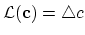and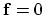in (2.1) is of special interest, since it represents the problems discussed in this work in its simplest form.

Using a backward Euler scheme and a finite element method with linear basic nodal functions we have the estimate [13,10,9],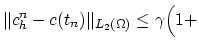ln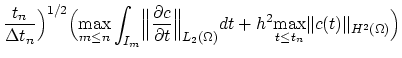(2.51)

for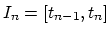and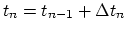, where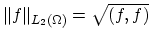, and(2.52)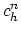is the finite element approximation at the discrete time step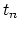, and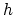is the the maximal edge length of the discretization. The estimate (2.51) is valid independently of the dimension of a given problem [13,10,9].

Taking into account that the logarithmic quantity in (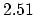) changes slowly, it can be absorbed in the constant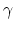and considering that,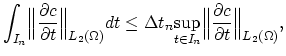(2.53)

we can write (2.51) as,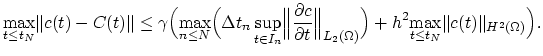(2.54)

The first term on the right side of (2.54) corresponds to the time discretization error and the second to the spatial discretization error .

If we want to achieve the time discretization error to be bounded by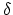for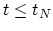, we have to ensure that,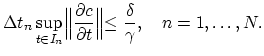(2.55)

Making the plausible assumption that,(2.56)

we obtain a rule for choosing the local time step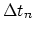. Starting with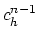we calculateusing the time step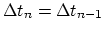. If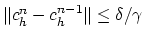, the valueis accepted and otherwiseis decreased. Variations of this approach are also possible. For example, the initialcan be chosen as ,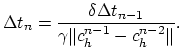(2.57)

To estimate the error of the spatial finite element discretization several methods are known today. These methods are based on the estimation of the second term on the right side of (2.54) using the solution obtained by means of the finite element method (a posteriori error estimators)[13,10,9].

First we will present the flux error estimator based on the work by Babuska, Bieterman, and Rheinboldt .

We consider a tethraedron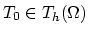and define a tehraedron-patch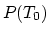as the subset of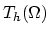of all tethraedrons which have one common face with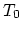. Each point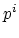of the discretization holds a concentration value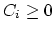and inside of the each element the concentration is linearly interpolated as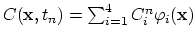. In the following we omit the discrete time index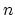, because we consider only a single time step.

The concentration gradient, calculated by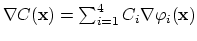, is constant on each element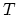and discontinuously changes between two neighboring elements. We write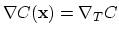for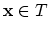. This discontinuity expresses the error of the approximation and can be quantified on the tehraedron-patchas the flux error estimator,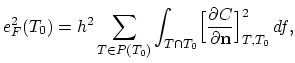(2.58)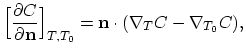(2.59)

where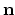is the outside normal on the face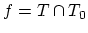andis the length of the longest edge of the tethraedron.

The second spatial discretization error estimator presented here is the gradient recovery error estimator which is widely used today in the finite element community .

For the derivation of the gradient recovery error estimator we first need the recovered gradient. The recovered gradient is calculated as the Lagrange interpolate at the nodes of the mesh. Taking a nodeand defining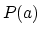as a point-patch of all elements which contain node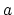as one of its nodes. The recovered gradient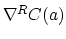at the node, is then calculated as,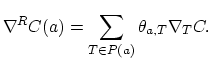(2.60)

We chose the weights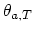of the Lagrange interpolate as,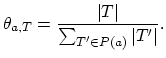(2.61)

The values of the concentration gradient on the element nodes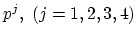enable to define a linear approximation of the gradient inside the element as,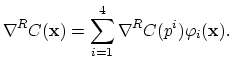(2.62)

The deviation of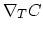from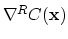on the elementgives the gradient recovery error estimator,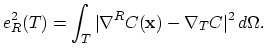(2.63)

Although the gradient recovery error estimator and the flux error estimator are apparantly crude and derived on the basis of a simple parabolic model problem, they result in a suprisingly good error estimate . These error estimators are used as indicators for the local mesh adaptation in several FEM based software tools [15,16,17].

For the applications discussed in this thesis the physically motivated dosis error estimator (Section 3.9) has even more importance than the error estimators presented above.

Independent of the actual mesh adaptation strategy and error estimator, the following algorithm for the finite element adaptive computation can be applied ,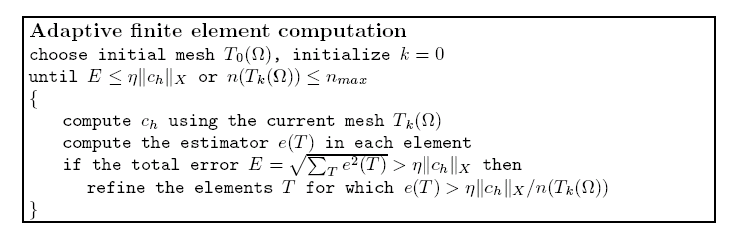Here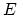denotes the total error,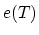is an error estimator,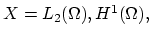or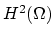, and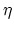is a given tolerance for the relative error.Next: 3. Diffusion Phenomena in Up: 2. Finite Element Method Previous: 2.8 General Solving Procedure

H. Ceric: Numerical Techniques in Modern TCAD